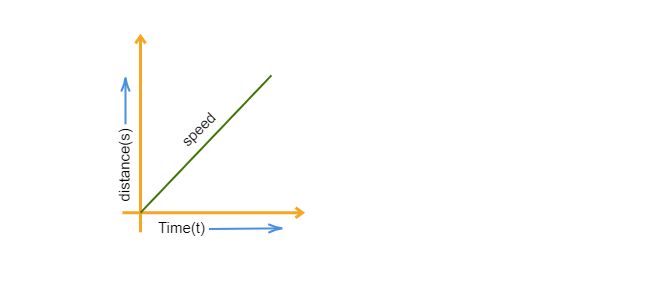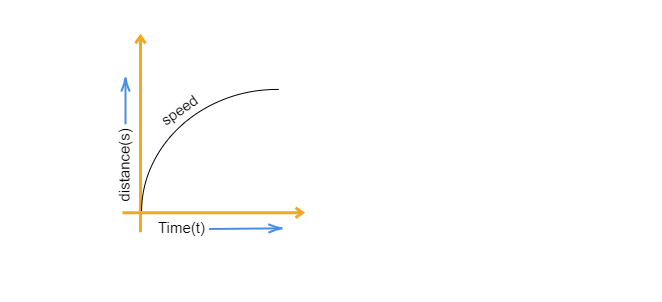# What is the nature of the distance-time graph for uniform and non-uniform motion of an object?

Distance-time graph for the uniform motion: The speed of a moving object in uniform motion will be constant. Therefore, the slope of the distance-time graph of an object in uniform motion will be a straight line.The distance-time graph for the non-uniform motion:  The slope of the distance-time graph for the non-uniform motion for a moving object is a curved line as its speed is not constant.Updated on: 10-Oct-2022

112 Views